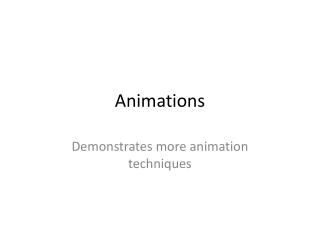DownloadDownload PresentationAnimations

# Animations

Download Presentation## Animations

- - - - - - - - - - - - - - - - - - - - - - - - - - - E N D - - - - - - - - - - - - - - - - - - - - - - - - - - -
##### Presentation Transcript

1. Animations Demonstrates more animation techniques

2. More Animation Examples • The Animations.zip program demonstrates additional animation methods. • The opening form, featuring the old Michigan Stadium (without luxury boxes or a losing team), demonstrates using an array of points to define a path for an object to follow. • The Shapes form demonstrates have to build a picture in layers (this technique would be simple to apply to the drawings you are creating for assignment 8). • The Velocity form demonstrates how to apply some simple physics (the constant downward acceleration of gravity) to objects. • The Multiball form demonstrates how to manage numerous objects at the same time.

3. The Punt Return • The punt return (frmAnimationExamples) uses a pre-defined set of points:

4. Punt Return • When the timer ticks, the ball moves to the next point on the curve:

5. Adding Some Physics • The Velocity and Multiball forms in the Animations example demonstrate incorporating some simple physics into a class. • The ball.vb class has a velocity variable (mv) which is a Structure consisting of x and y components. • Since these balls will be flying close to ground, they will obey the simplified law of gravity—gravity is a constant downward acceleration (g). The program assumes no air resistance, so the x velocity will remain constant.

6. Coding Gravity • Here’s the code: • Every tick of the timer, the object moves a distance equivalent to its velocity in each direction (assuming velocity is in units of pixels/tick). • The y velocity is then reduced by the value of gravity, so it will be less the next time the timer ticks. • By varying the x and y velocities and the value of gravity, we get different trajectories for the balls.

7. The football class • The football class inherits from the ball class, overriding only how it is drawn. • The constructor simply calls ball’s constructor (VB requires that you do this if you want a custom constructor in a derived class).